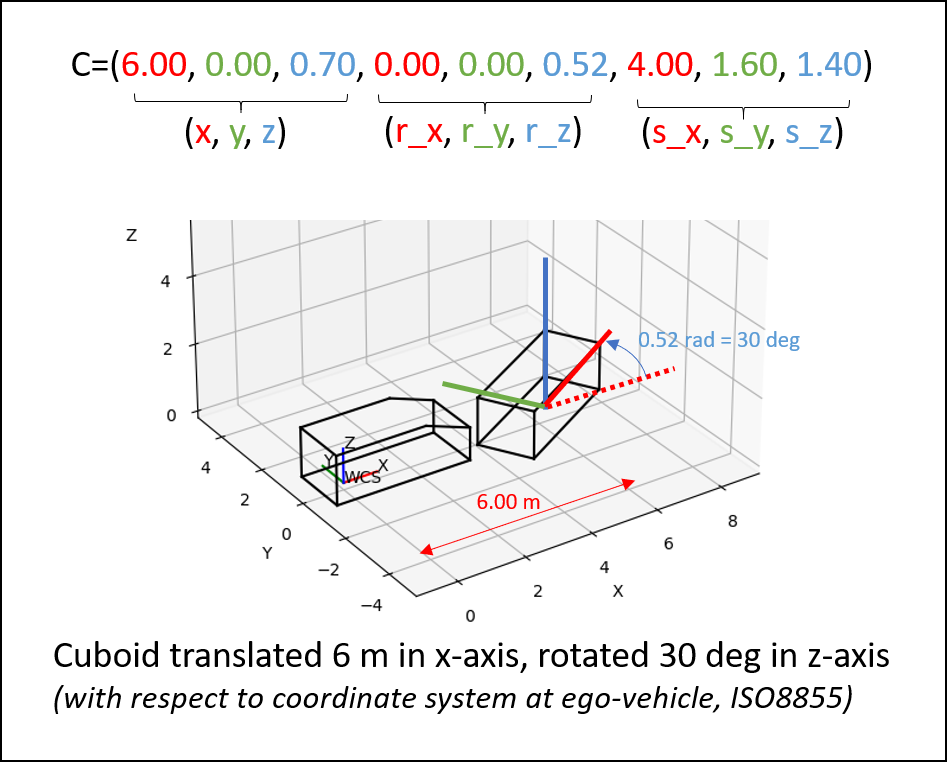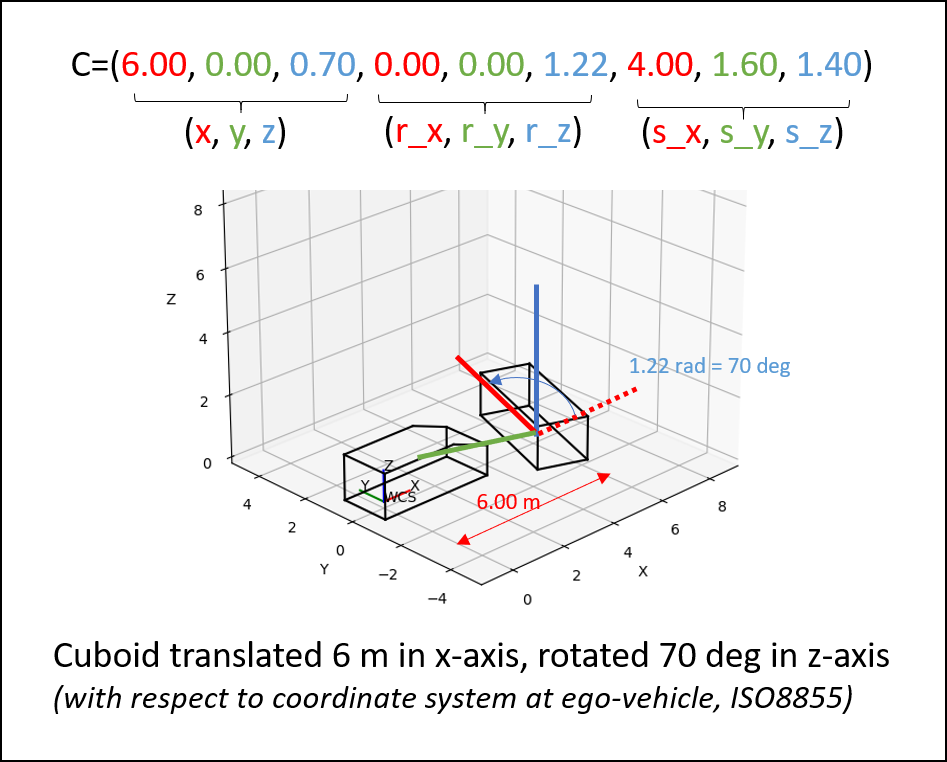## 1. Introduction

### 1.1. Foreword

OpenLABEL is a standard for annotation formats, which can be used for data streams and scenarios created during the development of automated driving features. For an automated vehicle to operate within its design domain a understanding of its surroundings is crucial. The vehicle senses its environment by using different sensors (e.g. cameras, lidar, radar). The data from the sensors must be interpreted to create a processable image of the world, based on this image the vehicle can choose its action. For the development of such features a ground truth is necessary. This ground truth is created by annotating the raw data. With in the data the following can be annotated:

• objects (traffic participants, static objects, etc.)

• relations between objects (pedestrian and bicycle)

• areas (e.g. free space, non-drivable areas)

• …​

When labeling an object it is possible to use different methods. On the one hand the labelling method depends on the data, but also on the use case. Labeling a vehicle in Lidar data requires a 3 dimensional method, while labeling the same car in a video image requires only a 2 dimensional method.

During the development of an automated vehicle it is very important to identify the scenarios that fit the use case and the design domain the vehicle is supposed to operate in. For that purpose it is useful to label scenarios according to their content and relevance to the project.

Developing an automated vehicle is a very complex tasks and usually many parties are involved in such a project. This makes an exchangeable format very valuable.

In the OpenLABEL concept project it is the goal of the project group to create concepts to establish a common format that can provide the industry with a standard for the annotation of sensor data and scenarios. The format will be machine processable and still be human readable. Also the group creates a basis to build a user guide. The user guide will explain to any future user how to use the available labeling methods, that are supported by OpenLABEL. The concept paper created in this project will serve as a basis for a follow up standard development project.

### 1.2. Overview

This concept paper serves as a source for a future development of the OpenLABEL standard. In the OpenLABEL Concept Project the basic concepts for a future annotation standard are created. To this the project has four different working groups. Each working group created their own concept and aligned them within the project and with the other OpenX Activities at ASAM.

The Concept project has four workpackages:

1. OpenLABEL Format

2. OpenLABEL User Guide for Labeling methods

3. OpenLABEL Taxonomy as Interface for OpenXOntology

4. OpenLABEL Scenario Labeling

### 1.3. Relation to other Standards

The OpenLABEL Concept has a close link to the OpenXOntology. This link will ensure that the OpenLABEL Taxonomy requirements are met in the upcoming OpenX Core Domain Model.

Relation to other Standards:

• ASAM OpenDRIVE

• ASAM OpenSCENARIO

• ASAM OSI

• ASAM OpenXOntology (Standard is still under work)

• ASAM OpenODD

• BSI PAS 1883

## 2. Annotation Format

### 2.1. Introduction annotation format

This section details the annotation format of OpenLABEL. The format is key to make OpenLABEL flexible enough to host different labeling use cases, ranging from simple object-level annotation in images (e.g. with bounding boxes), to complex multi-stream labeling of scene semantics (e.g. with actions, relations, contexts). The annotation format is then understood as the materialization of labels in files or messages, that can be stored or exchanged between machines. The format shall address a number of requirements:

• Different scene elements (objects, actions, contexts, events)

• Temporal description of elements (with frames and timestamps)

• Hierarchical structures, with nested attributes

• Semantic relations between elements (e.g. object performing an action)

• Multiple source information (i.e. multi-sensor)

• Preservation of identities of elements through time

• Encoding mechanisms to represent different geometries (e.g. bounding boxes, cuboids, pixel-level segmentation, polygons, etc.)

• Enable linkage to ontologies and knowledge repositories

• Ability to update annotations in online processes (extensible)

• Scalable and searchable (good traceability properties)

The annotation format management shall also define which properties are mandatory and which optional, types of variables, and serialization mechanisms to create persistent content, such as files or messages.

Next sections detail all these aspects, with examples and definitions.

### 2.2. JSON schema

One modern approach to define data structure is to create a JSON schema, which is itself a JSON document that contains descriptions and constraints on the structure and content of JSON files, and it also provides a data model.

A JSON schema can be used to validate the content of a JSON file, guaranteeing that the file follows the constraints and structure dictated by the schema. Also, schemas can be used by programming languages to create object-oriented structures which facilitate manipulation, edition and access to information of a JSON file.

The annotation format of OpenLABEL is then proposed to be hosted on a detailed JSON schema file, and as a consequence, annotation files will be JSON files following the schema. Appended to this document the draft openlabel_schema_json-v1.0.0.json can be found.

### 2.3. Structure of the OpenLABEL format

In OpenLABEL, a scene can be either a subset of the reality that needs to be described for further analysis, or a virtual situation that needs to be materialized. In the former case, reality is typically perceived by sensors, which get discrete measures of magnitudes from the scene at a certain frequency. In the latter, sensors can be ignored, and the scene described by its components and logical sequence.

Several concepts conform the basis of the OpenLABEL format. As it will be shown, these pieces constitute the foundations to create rich descriptions of scenes, either as an entire block (e.g. serialized as a file), or frame-by-frame (e.g. serialized as message strings).

• Elements: objects, actions, events, contexts and relations that compose the scene, each of them with an integer unique identifier for the entire scene.

• Frames: discrete containers of information of Elements and Streams for a specific time instant.

• Streams: information about the discrete data sources (e.g. coming from sensors), to describe how reality is perceived at each stream (e.g. with intrinsics/extrinsics of cameras, timestamps from sensors, etc.).

• Coordinate Systems: the spatial information that defines the labeled geometries refer to specific coordinate systems, which can be defined and labeled themselves within OpenLABEL. Transforms between coordinate systems determine how geometries can be projected from one reference to another (e.g. from one sensor to a static reference, or because of odometry entries.) See coordinate systems section.

• Metadata: descriptive information about the format version, file version, annotator, name of the file, and any other administrative information about the annotation file.

• Ontologies: pointers to knowledge repositories (URLs of ontologies) that are used in the annotation file. Elements labeled can point to concepts at these ontologies, so a consuming application can consult the element meaning or investigate additional properties.

The basic serialization of an OpenLABEL JSON string (prettified), with just administrative information and versioning is:

 1
2
3
4
5
6
7
8
9
10
{
"openlabel": {
"annotator": "John Smith",
"file_version": "0.1.0",
"schema_version": "1.0.0",
"comment": "Annotation file produced manually",
}
}
}


Next subsections show how to add different concepts (Elements, Frames and Streams) and show examples for relevant use cases considered in this concept-paper. For the sake of space and readability, partially collapsed JSON strings will be shown.

#### 2.3.1. Elements

Elements is the name for Objects, Actions, Events, Contexts and Relations, which are all treated similarly within the OpenLABEL format, in terms of properties, types and hierarchies.

Elements have a name, a unique identifier, a semantic type, and an ontology identifier.

• name: this is a friendly identifier of the Element, not unique, but serves for human users to rapidly identify Elements in the scene (e.g. "Peter").

• uid: this is a unique identifier which determines the identity of the Element. It can be a simple unsigned integer (from 0 upwards, e.g. "0"), or a Universal Unique Identifier (UUID) of 32 hexadecimal characters (e.g. "123e4567-e89b-12d3-a456-426614174000").

• type: this is the semantic type of the Element. It determines to which class the Element belongs to (e.g. "Car", "Running").

• ontology id: this is the identifier (in the form of a unsigned integer) of the ontology URL which contains the full description of the class referred as the semantic type. See Ontologies.

Next subsections show the purpose of each of the Element types.

##### Objects

Objects are the main placeholders of information about physical entities in scenes. Examples of Objects are pedestrians, cars, the ego-vehicle, traffic signs, lane markings, building, trees, etc.

An Object in OpenLABEL is defined by its name, type, and indexed inside the annotation file by an integer unique identifier:

 1
2
3
4
5
6
7
8
9
10
11
12
13
14
15
16
17
18
19
20
21
22
23
24
{
"openlabel": {
...
"objects": {
"1": {
"name": "van1",
"type": "Van"
},
"2": {
"name": "cyclist2",
"type": "Cyclist"
},
...
"16": {
"name": "Ego-vehicle",
"type": "Car"
},
"17": {
}
}
}
}


When using UUIDs, the keys are substituted by 32 hexadecimal character strings:

 1
2
3
4
5
6
7
8
9
10
11
{
"openlabel": {
...
"objects": {
"c44c1fc2-ee48-4b17-a20e-829de9be1141": {
"name": "van1",
"type": "Van"
},
}
}
}

 unique identifiers need not to be sequential nor start at 0, which is useful to preserve identifiers from other label files. They only need to be unique for each element type. Each element type (action, object, event, context and relation) has its own list of unique identifiers.
 name and type are mandatory fields according to the JSON schema. However, they can be left empty as they are not used to index. In general, name can be used as a friendly descriptor, while type refers to the semantic category of the element (see more about semantics in Ontologies).
 JSON only permits keys to be strings. Therefore, the integer unique identifiers are converted to strings, "0". Though, carefully written APIs can parse JSON strings into integers for better access efficiency and sorting capabilities.

In addition, some Objects can be defined for certain sets of frame intervals, while others are left frame-less.

 1
2
3
4
5
6
7
8
9
10
11
12
13
14
15
16
17
18
19
20
21
{
"openlabel": {
...
"objects": {
"1": {
"name": "van1",
"type": "Van"
"frame_intervals": [{
"frame_start": 0,
"frame_end": 10
}
]
},
...
"16": {
"name": "Ego-vehicle",
"type": "Car",
},
}
}
}

 Frame intervals are represented as an array, as the same Object might appear and disappear from the scene, and thus be represented by several frame intervals.

When Objects are defined with such time information, entries of them are added to Frames.

##### Actions, Events, Contexts

Almost completely analogous to Objects, other elements defined in OpenLABEL are Actions, Events and Contexts.

• Action: a description of a semantically meaningful situation. It can be defined for several frame intervals (just like Objects). E.g. "isWalking".

• Event: a single instant in time which has a semantic load, and that typically triggers other Events or Actions, e.g. "startsWalking".

• Context: any other descriptive information about the scene that has either not spatial or temporal information, or does not suit well under the term Action or Event. For instance, Context can refer to properties of the scene (e.g. "Urban", "Highway"), weather conditions (e.g. "Sunny", "Cloudy"), general information about the location (e.g. "Germany", "Spain"), or any other relevant tag.

These elements are included into the OpenLABEL JSON structure just like Objects are:

 1
2
3
4
5
6
7
8
9
10
11
12
13
14
15
16
17
18
19
20
21
22
23
24
25
{
"openlabel": {
...
"actions": {
"0": {
"name": "following1",
"type": "following",
"frame_intervals": [{"frame_start": 0, "frame_end": 10}]
}
},
"events": {
"0": {
"name": "crossing1",
"type": "startsCrossing",
"frame_intervals": [{"frame_start": 5, "frame_end": 5}]
}
},
"contexts": {
"0": {
"name": "",
"type": "Urban"
}
}
}
}

 In the example above, the Context is defined frame-less, and as so, assumed to exist or be valid for the entire scene. As there are other elements (e.g. actions) with defined frame intervals, this Context also appears in all defined Frames.
 Contexts can have frame intervals defined, as contextual information may vary through time (e.g. a scene starts in a Urban environment and them ends within a highway).
##### Relations

Relations are elements used to define relations between other elements. Though represented just like any other element within the OpenLABEL JSON schema, i.e. with name, type, defined with static and dynamic information, they have special features as they are foundational elements that allow advanced semantic labeling.

A Relation is defined as an RDF triple subject-predicate-object. The predicate can be seen as the edge connecting the two vertices (subject and object), if we imagine the triple as a graph.

The predicate is labeled as the Relation's type, while the subject and object are added as rdf_subjects and rdf_objects respectively. In OpenLABEL, rdf_objects and rdf_subjects are pointers to other defined elements in the scene, for instance an Object, a Context, Event or Action.

The predicate itself determines what is the relation between these elements. It is possible to define the relation with free text, using terms like "isNear", "belongsTo", "isActorOf", but, in general, it is a recommended practice to use the ontology_uid property to use relation concepts well defined in a domain model.

Although an RDF triple strictly defines a connection between one object and one subject, in OpenLABEL it is possible to define multiple rdf_subjects and rdf_objects for the same predicate/relation. This feature is useful for compositional relations such as "isPartOf".

 1
2
3
4
5
6
7
8
9
10
11
12
13
14
15
16
17
18
19
20
21
22
23
24
25
26
27
28
29
30
31
32
33
34
35
{
"openlabel": {
...
"objects": {
"0": {
"name": "car0",
"type": "#Car",
"ontology_uid": 0
},
...
},
"actions": {
"0": {
"name": "",
"type": "#isWalking",
}
},
"relations": {
"0" : {
"name" : "",
"type" : "isSubjectOfAction",
"rdf_subjects" : [{
"uid" : 0,
"type" : "object"
}
],
"rdf_objects" : [{
"uid" : 0,
"type" : "action"
}
]
},
}
}
}


Describing scenes can be done by decomposing a high-level description into atomic triples that can then be written formally in an OpenLABEL JSON file.

*Relation*s provide complete flexibility to represent any kind of linkage between other Elements (including Objects, Actions, Events, Contexts, and even Relations).

How to represent each particular case is left to the user of OpenLABEL. A typical, and recommended practice for transitive actions is as follows: a transitive action (an action with a subject and an object) can be added using two RDF triplets, one defining the subject of the action, with "isSubjectOfAction" and another defining the object of the action, with "isObjectOfAction".

 The terms object (and subject) in RDF language need not to be confused with the term Object in OpenLABEL.

Let’s consider the following example:

Ego-vehicle follows cyclist

It can be decoupled into two RDFs triples:

Ego-vehicle isSubjectOfAction follows and cyclist isObjectOfAction follows

This pair of RDF triples are way easier to manage from an ontology point of view, and also in graphical databases implementations, since this way, not only the physical objects (Ego-vehicle and cyclist), but also the action itself (Follows) are defined as concepts (classes) in the ontology, and thus have properties, and be part of a hierarchy of classes. Whereas the edges (links or relations) are left as isSubjectOfAction, and isObjectOfAction. Other possible useful relations are: isPartOf, sameAs, hasAttribute, and other spatio-temporal relations, such as isNear, happensBefore, etc. Most of this discussion is inherited from ongoing discussions in the OpenXOntology project.

#### 2.3.2. Frames

Dynamic information about elements is stored within the corresponding Frames. Each frame is indexed within the OpenLABEL file with an integer unique identifier exactly as elements are.

Each frame contain structures of elements with only the non-static information, i.e. name, type and other static structures are ommitted.

 1
2
3
4
5
6
7
8
9
10
11
12
13
14
15
16
17
18
19
20
{
"openlabel": {
...
"frames": {
"0": {
"objects": {
"1": {}
}
}
},
"objects": {
"1": {
"name": "",
"type": "Van",
"frame_intervals": [{"frame_start": 0, "frame_end": 10}]
},
...
}
}
}


If the specific information of the Object for a given frame is nothing but its existence, then, the Object's information at such frame is just a pointer to its unique identifier.

When frame-specific information is added, it is enclosed inside the corresponding frame and object:

 1
2
3
4
5
6
7
8
9
10
11
12
13
14
15
16
17
18
19
20
21
{
"openlabel": {
...
"frames": {
"0": {
"objects": {
"1": {
"object_data": {
"bbox": [{
"name": "shape",
"val": [12, 867, 600, 460]
}
]
}
}
}
}
}
...
}
}

 More information about the "object_data" structure of the example above is discussed in Element data.

A Frame can also contain information about its own properties, such as its timestamp. In general terms, a Frame shall be seen as the container of information corresponding to a single instant. Synchronization information for multiple streams can also be labeled in order to precisely define which annotations correspond to what instant and from which sensor (see Streams).

 1
2
3
4
5
6
7
8
9
10
11
12
13
{
"openlabel": {
...
"frames": {
"0": {
"objects": { ... },
"frame_properties": {
"timestamp": "2020-04-11 12:00:01"
}
}
}
}
}


Even when only pointers are present within a Frame, this structure ensures:

• Frames can be serialized independently and sent via messaging to other computers or systems online

• Efficient access to static information using pointers, and avoiding repetition of static information

The union of frame intervals of all elements in the scene define the frame intervals of the annotation file itself:

 1
2
3
4
5
6
7
8
9
10
11
12
13
14
15
16
17
18
{
"openlabel": {
...
"frame_intervals": [{
"frame_start": 0,
"frame_end": 150
},{
"frame_start": 160,
"frame_end": 180
}
],
"frames": {
"0": { ... },
"1": { ... },
...
}
}
}


Then, frame_intervals define which frames exist for this annotation file.

#### 2.3.3. Ontologies

The OpenLABEL JSON schema defines the allowed names of the "keys" of the key-value pairs of the JSON file. And also the expected type, structure and format of the "values" (in some minor cases also the allowed values, specially for strings).

However, in most cases, the provision of meaning of the "values" is left free for the annotator. For instance, the type of an Object can be declared as Person, while other annotator might choose Pedestrian if the labeling tool imposes no restrictions.

OpenLABEL provides a door to link to ontologies, as representations of the domain-model of interest. This is achieved labeling the ontologies and the ontology_uid for elements:

 1
2
3
4
5
6
7
8
9
10
11
12
13
14
15
16
17
18
19
20
21
{
"openlabel": {
...
"ontologies": {
"0": "http://www.somedomain.org/ontology",
"1": "http://www.someotherdomain.org/ontology"
},
"objects": {
"0": {
"name": "car0",
"type": "#Car",
"ontology_uid": 0
},
"1": {
"name": "person1",
"type": "#Person",
"ontology_uid": 1
}
}
}
}


The object’s type can then be read as the concatenation of the url of the ontology pointed out by the ontology_uid and the type entry of the object. Labeling tools might provide the ability to parse the ontologies (either remote or local) and offer the annotator a list of options, suggestions, or translation capabilities.

Also, the numbers used to describe some geometries, such as cuboid require that the same consensus and criteria is maintained and guaranteed by the standard. As a consequence, a default ontology for OpenLABEL is assumed to exist, to be aligned with the OpenXOntology project, where all the terms used in the OpenLABEL JSON schema are defined.

#### 2.3.4. Relations

Relations are elements used to define relations between other elements. Though represented just like any other element within the OpenLABEL JSON schema, i.e. with name, type, defined with static and dynamic information, they have special features as they are foundational elements that allow advanced semantic labeling.

A Relation is defined as an RDF triple subject-predicate-object. The predicate can be seen as the edge connecting the two vertices (subject and object), if we imagine the triple as a graph.

The predicate is labeled as the Relation's type, while the subject and object are added as rdf_subjects and rdf_objects respectively. In OpenLABEL, rdf_objects and rdf_subjects are pointers to other defined elements in the scene, for instance an Object, a Context, Event or Action.

The predicate itself determines what is the relation between these elements. It is possible to define the relation with free text, using terms like "isNear", "belongsTo", "isActorOf", but, in general, it is a recommended practice to use the ontology_uid property to use relation concepts well defined in a domain model.

Although an RDF triple strictly defines a connection between one object and one subject, in OpenLABEL it is possible to define multiple rdf_subjects and rdf_objects for the same predicate/relation. This feature is useful for compositional relations such as "isPartOf".

 1
2
3
4
5
6
7
8
9
10
11
12
13
14
15
16
17
18
19
20
21
22
23
24
25
26
27
28
29
30
31
32
33
34
35
{
"openlabel": {
...
"objects": {
"0": {
"name": "car0",
"type": "#Car",
"ontology_uid": 0
},
...
},
"actions": {
"0": {
"name": "",
"type": "#isWalking",
}
},
"relations": {
"0" : {
"name" : "",
"type" : "isSubjectOfAction",
"rdf_subjects" : [{
"uid" : 0,
"type" : "object"
}
],
"rdf_objects" : [{
"uid" : 0,
"type" : "action"
}
]
},
}
}
}


Describing scenes can be done by decomposing a high-level description into atomic triples that can then be written formally in an OpenLABEL JSON file.

TODO: add examples of transitive maneuvers

 The terms object (and subject) in RDF language need not to be confused with the term Object in OpenLABEL.

#### 2.3.5. Element data

The OpenLABEL JSON schema defines the possibility to nest element data within elements. For instance, object_data can be embedded inside Objects, action_data inside Actions, and event_data and context_data inside Events and Contexts, respectively.

This gives the ability to describe to great level of detail any aspect of the elements. On the one hand, element-level descriptions, as those defined in sections above, provide the ability to describe intrinsic, high-level information about objects, actions, etc. On the other hand, element data-level information can be used to add how the elements are perceived by sensors, or details about their geometry or any other relevant aspect.

Since the general structure defines equivalent hierarchies for elements at the root and inside each frame, element data can then be naturally defined statically (time-less), or dynamically (for specific frame intervals).

The OpenLABEL JSON schema defines a comprehensive list of primitives that can be used to encode element data information. Some of them are completely generic, such as text, num or boolean, while others are specific to geometric magnitudes, like poly2d, cuboid, etc.

The list of currently supported element data is:

• boolean: true or false

• num: a number (can be integer or floating)

• text: a string of chars

• vec: a vector or array of numbers

• bbox: a 2D bounding box

• rbbox: a 2D rotated bounding box

• binary: a binary content stringified to base64

• cuboid: a 3D cuboid

• image: an image payload encoded and stringified to base64

• mat: a NxM matrix

• point2d: a point in 2D space

• point3d: a point in 3D space

• poly2d: a 2D polygon defined by a sequence of 2D points

• poly3d: a 3D polygon defined by a sequence of 3D points

• area_reference: a reference to an area

• line_reference: a reference to a line

• mesh: a 3D mesh of points, vertex and areas

See the OpenLABEL JSON schema for details on each of them.

One interesting distinction is that the first four element data types of the list (boolean, num, text, vec) are defined as non-geometric, and thus can be themselves being nested within other geometric element data.

 1
2
3
4
5
6
7
8
9
10
11
12
13
14
15
16
17
18
19
20
21
22
23
24
25
26
27
28
29
30
31
32
33
34
35
36
37
38
39
40
41
42
43
44
45
46
{
"openlabel": {
...
"frame_intervals": [ ... ],
"frames": {
"0": {
"objects": {
"0": {
"object_data": {
"bbox": [{
"name": "shape",
"val": [300, 200, 50, 100]
"attributes": {
"boolean": [{
"name": "visible",
"val": true
}
]
}
},{
"val": [250, 200, 100, 200]
}
]
}
}
}
}
},
...
"objects": {
"0": {
"name": "car0",
"type": "car",
"frame_intervals": [{"frame_start": 0, "frame_end": 0}],
"object_data": {
"text": [{
"name": "color",
"val": "blue"
}
],
}
}
}
}
}


The same concept applies to action_data, event_data and context_data, with the main different that they can not have geometric element data inside (e.g. bbox, cuboid, etc.), but only non-geometric types such as text, vec, num and boolean.

Full detail of the inner structure of each of these element types is provided in Element Data types.

Since element data is not indexed by integer unique identifiers like elements, the structure defines a mechanism to have an index over each element element data by adding element data pointers. For instance, object_data_pointers within an Object contain key-value pairs to identify which object_data names are used, and which are their associated frame_intervals.

 1
2
3
4
5
6
7
8
9
10
11
12
13
14
15
16
17
18
19
20
21
22
23
24
25
26
27
28
29
30
31
{
"openlabel": {
...
"objects": {
"0": {
"name": "car0",
"type": "car",
"frame_intervals": [{"frame_start": 0, "frame_end": 0}],
"object_data": {
"text": [{
"name": "color",
"val": "blue"
}
],
},
"object_data_pointers": {
"color": {
"type": "text",
},
"shape": {
"type": "bbox",
"frame_intervals": [{"frame_start": 0, "frame_end": 0}],
"attributes": {
"visible": "boolean"
}
}
}
}
}
}
}


As can be seen from the example above, the pointers refer to both static (frame-less) and dynamic (frame-specific) object_data, and also contain information about the nested attributes. In practice, this feature is extremelly useful for fast retrieval of element data information from the JSON file, without the need to explore the entire set of frames.

#### 2.3.6. Streams

Complex scenes may be observed by several sensing devices, and thus producing multiple streams of data. Each of these streams might have different properties, intrinsic and extrinsic information, and frequency. The OpenLABEL JSON schema defines the possibility to specify such information for a multi-sensor (and thus, a multi-stream) set-up, by allocating space for such metadata descriptions, and the ability to specific, for each labeled element, what stream they correspond to.

 1
2
3
4
5
6
7
8
9
10
11
12
13
14
15
16
17
18
19
20
21
22
23
24
25
26
27
28
29
30
31
32
33
34
35
36
37
38
39
40
41
42
43
44
45
46
{
"openlabel": {
...
"streams": {
"Camera1": {
"type": "camera",
"description": "Frontal camera from vendor X",
"stream_properties": {
"intrinsics_pinhole": {
"camera_matrix_3x4": [ 1000.0,    0.0, 500.0, 0.0,
0.0, 1000.0, 500.0, 0.0,
0.0,    0.0,   0.0, 1.0],
"distortion_coeffs_1xN": [],
"height_px": 480,
"width_px": 640
},
}
}
}
},
...
"frame_properties": {
"streams": {
"Camera1": {
"stream_properties": {
"intrinsics_pinhole": {
"camera_matrix_3x4": [ 1000.0,    0.0, 500.0, 0.0,
0.0, 1000.0, 500.0, 0.0,
0.0,    0.0,   0.0, 1.0],
"distortion_coeffs_1xN": [],
"height_px": 480,
"width_px": 640
},
"sync": {
"frame_stream": 1,
"timestamp": "2020-04-11 12:00:02"
}
}
}
},
"timestamp": "2020-04-11 12:00:01"
}
}
}

 As shown in the example, stream_properties can be defined either within the static part (i.e. inside the "metadata/streams" field), or frame-specific, inside the "streams" field of a given frame.
 The sync field within stream_properties can define the frame number of this stream that corresponds to this frame, along with timestamping information if needed. This feature is extremelly handy to enable the annotation of multiple cameras which might not be perfectly aligned. In such case, frame 0 of the annotation file corresponds to frame 0 of the first stream to occurr. In general, frame_stream identifies which frame of this stream corresponds to the frame in which it is enclosed.

To specify that a certain object data information corresponds to a certain stream, the OpenLABEL JSON schema defines the property stream for both elements and element data:

 1
2
3
4
5
6
7
8
9
10
11
12
13
14
15
16
17
18
19
20
21
22
23
24
25
26
27
28
{
"openlabel": {
"frames": {
"0": {
"objects": {
"0": {
"object_data": {
"bbox": [{
"val": [600, 500, 100, 200],
"stream": "Camera1"
}
]
}
}
}
}
},
...
"objects": {
"0": {
"name": "",
"type": "Person",
"stream": "Camera1"
}
}
}
}


#### 2.3.7. Coordinate Systems and Transforms

As described in the coordinate system section, labels can be defined as relative to specific coordinate systems. This is particularly necessary for geometric labels, such as polygons, cuboids or bounding boxes, which define magnitudes under a certain coordinate system. For instance, a 2D line can be defined within the coordinate system of an image frame, and a 3D cuboid inside a 3D Cartesian coordinate system.

In addition, as multiple coordinate systems can be defined, it is necessary to define as well mechanisms to declare how to convert values of magnitudes from one coordinate system to another. Therefore, Transforms, between two coordinate systems are also defined.

Coordinate systems can be declared with a friendly name, used as index, and in the form of parent-child links, to establish their hierarchy:

• type: the type of coordinate system is defined so reading applications have a simplified view of the hierarchy: can be scene_cs (this corresponds to static coordinate system), local_cs (this is a coordinate system of a rigid body moving sensors), sensor_cs (a coordinate system attached to a sensor) or custom_cs (any other coordinate system defined by the user).

• parent: despite the type of coordinate system defined, each coordinate system can declare its parent coordinate system in the hierarchy.

• pose_wrt_parent: a default or static pose of this coordinate system with respect to the declared parent. Can be set in the form of a 4x4 matrix enclosing a 3D rotation and 3D translation.

• children: the list of children for this coordinate system.

 1
2
3
4
5
6
7
8
9
10
11
12
13
14
15
16
17
18
19
20
21
22
23
24
25
26
27
28
29
30
31
32
33
34
35
36
37
{
"openlabel": {
...
"coordinate_systems": {
"odom": {
"type": "scene_cs",
"parent": "",
"pose_wrt_parent": [],
"children": [
"vehicle-iso8855"
]
},
"vehicle-iso8855": {
"type": "local_cs",
"parent": "odom",
"pose_wrt_parent": [1.0,0.0,0.0,0.0,0.0,1.0,0.0,0.0,0.0,0.0,1.0,0.0,0.0,0.0,0.0,1.0],
"children": [
"Camera1",
"Camera2"
]
},
"Camera1": {
"type": "sensor_cs",
"parent": "vehicle-iso8855",
"pose_wrt_parent": [1.0,0.0,0.0,0.0,0.0,1.0,0.0,0.0,0.0,0.0,1.0,0.0,0.0,0.0,0.0,1.0],
"children": []
},
"Camera2": {
"type": "sensor_cs",
"parent": "vehicle-iso8855",
"pose_wrt_parent": [1.0,0.0,0.0,0.0,0.0,1.0,0.0,0.0,0.0,0.0,1.0,0.0,0.0,0.0,0.0,1.0],
"children": []
}
},
...
}
}


Of course, the transforms between coordinate systems can also be defined for each frame, overriding the default-static pose defined above. Transforms are defined with a friendly name used as index, and the following properties:

• src: the name of the source coordinate system, whose magnitudes the transform converts into destination coordinate system. This must be the name of a valid (declared) coordinate system.

• dst: the destination coordinate system in which the source magnitudes are converted after applying the transform. This must be the name of a valid (declared) coordinate system.

• transform_src_to_dst: this is the transform expressed in algebraic form, for instance as a 4x4 matrix enclosing a 3D rotation and a 3D translation between the coordinate systems.

• additional properties: as most elements in the OpenLabel format, it is also possible to add customized content as additional properties.

 1
2
3
4
5
6
7
8
9
10
11
12
13
14
15
16
17
18
19
{
"openlabel": {
...
"frames": {
"2": {
"frame_properties": {
"transforms": {
"vehicle-iso8855_to_Camera1": {
"src": "vehicle-iso8855",
"dst": "Camera1",
"transform_src_to_dst": [[1.0,0.0,0.0,0.0,0.0,1.0,0.0,0.0,0.0,0.0,1.0,0.0,0.0,0.0,0.0,1.0]]
}
}
}
}
}
...
}
}

 For non-3D coordinate systems, or with non-linear transforms, the format still applies, as long as the transform_src_to_dst is an array of numbers which contain all the necessary parameters to express the transform.
 In the example above, the destination coordinate system of the transform is Camera1 which is also the friendly name of a Stream. Indeed, Stream, which describe typically a sensor, such as a Camera or a LIDAR, should have associated coordinate systems, to defined their extrinsics or pose with respect to other coordinate systems, such as the ego-vehicle ISO8855 origin. Internal processes, such as intrinsic parameters or distortion coefficients (for pinhole or fisheye cameras) are defined inside the Stream fields as shown in Streams.

With this structure, it is possible to describe particular and typical transform cases, such as odometry entries:

 1
2
3
4
5
6
7
8
9
10
11
12
13
14
15
16
17
18
19
20
21
{
"openlabel": {
...
"frames": {
"0": {
"frame_properties": {
"transforms": {
"odom_to_vehicle-iso8855": {
"src": "odom",
"dst": "vehicle-iso8855",
"transform_src_to_dst": [1.0, 3.7088687554289227e-17, ...]
},
"raw_gps_data": [49.011212804408,8.4228850417969, ...],
"status": "interpolated"
}
}
}
}
...
}
}

 Using additional properties it is possible to embedd detailed and customized information about the transforms, such as additional non-linear coefficients, etc (in the example above, the raw gps entries are labeled for completeness).

### 2.4. Data types

This section provides details of the on the object_data primitives defined in OpenLABEL annotation format. Most of them are self-explanatory, as they represent primitives types like string, num (single number, floating point precision), vec (array of numbers), bool (boolean).

Geometric types are more complex. Next sub-sections describe their format.

#### 2.4.1. Bounding box: bbox

The 2D bounding box is defined as in section 2. It is defined as a array of 4 floating point numbers that define the center of the rectangle, and its width and height.

Thus, in the JSON schema file, a bounding box is defined as:

And example bounding box entry serialized in JSON is:

1
2
3
4
"bbox": {
"val": [400, 200, 100, 120]
},


Which means the center of the rectangle is the point (x, y)=(400, 200), while its dimensions are width=100, and height=120.

For complex set-ups it is possible to defined the coordinate_system these magnitudes are expressed with respect to. Also, it is possible to embed non-geometric object data inside:

 1
2
3
4
5
6
7
8
9
10
11
12
13
14
15
"bbox": {
"val": [400, 200, 100, 120],
"coordinate_system": "Camera1",
"attributes" : {
"boolean" : [{
"name" : "visible",
"val" : false
}, {
"name" : "occluded",
"val" : false
}
]
}
},


#### 2.4.2. Cuboid: cuboid

The 3D bounding box or cuboid is defined as in bounding box section. It is defined as a array of 10 floating point numbers that define the center of the rectangle (x, y, z), and its pose, as a quaternion vector (a, b, c, d) plus a dimensions vector (sx, sy, sz).

An example cuboid:

1
2
3
4
"cuboid": {
"name": "shape",
"val": [12.0, 20.0, 0.0, 1.0, 1.0, 1.0, 1.0, 4.0, 2.0, 1.5]
},


#### 2.4.3. Semantic segmentation: image and poly2d

Semantic segmentation responds to the need to define one or more labels per pixel of a given image (see semantic segmentation for details about the different possible use cases).

In terms of data format, such dense information can be tackled with different approaches, each of them having different purposes or responding to different needs:

• Separate images: historically, semantic segmentation information has been stored as separate images, usually formatted as PNG images (lossless). This is possibly the simplest approach, and the one offering the smallest storage footprint, at the cost of the need to manage separate files in the file system. Therefore, the main OpenLabel JSON file may contain the URL/URIs of these images (one or many):

 1
2
3
4
5
6
7
8
9
10
11
12
13
14
15
16
17
"objects": {
"0": {
"name": "",
"type": "",
"object_data": {
"string": [
{
"name": "semantic mask uri - dictionary 1",
"val": "/someURLorURI/someImageName1.png"
},{
"name": "semantic mask uri - dictionary 2",
"val": "/someURLorURI/someImageName2.png"
}
]
}
}
},

• Embedded images: image content can be expressed in base64 and then embedded within the JSON file. This approach will create largest JSON files (base64 adds 4/3 overhead) but alleviates the need to manage multiple files:

 1
2
3
4
5
6
7
8
9
10
11
12
13
14
15
16
"objects": {
"0": {
"name": "",
"type": "",
"object_data": {
"image": [
{
"name": "semantic mask - dictionary 1",
"mime_type": "image/png",
"encoding": "base64"
}
]
}
}
},

• Polygons: another option is to decompose the entire semantic segmentation mask into their inner pieces corresponding to the different classes or object instances. This approach has the benefit of identifying individual objects directly within the JSON file. Thus, a user application can directly read specific objects, without the need to load the PNG image and find the object of interest. The con is the increased JSON size. Polygons (2D) can be expressed directly as lists of (x,y) coordinates. However this may create very large and redundant information. Lossless compression mechanisms (e.g. RLE or Chain Code algorithms; in the example below, we are using the algorithm SRF6DCC, a reference implementation of this and other algorithms will be provided during the standardisation project of OpenLabel) can be applied, to convert the (possibly long) list of (x,y) coordinates into smaller strings:

 1
2
3
4
5
6
7
8
9
10
11
12
13
14
15
16
17
18
19
20
21
"objects": {
"0": {
"name": "car1",
"type": "#Car",
"object_data": {
"poly2d": [
{
"name": "poly1",
"val": ["5","5","1","mBIIOIII"],
"mode": "MODE_POLY2D_SRF6DCC",
"closed": false
}, {
"name": "poly2",
"val": [5,5,10,5,11,6,11,8,9,10,5,10,3,8,3,6,4,5],
"mode": "MODE_POLY2D_ABSOLUTE",
"closed": false
}
]
},
}
}

NOTE: Using polygons then implies that labels are created at object-level, rather than image-level, which might be extremelly useful for searching applications, which may be interesting in locating all objects of type car.
NOTE: Using PNG masks (either as separate files or embedded inside the JSON file) is definitely the preferred way to store labels for machine learning applications, which don't search inside the masks, but rather fed them directly into training pipelines.

### 2.5. Frames and Streams Synchronization

This section provides detail on the synchronization of multiple streams and their time information frames.

Labels can be produced to be related to specific streams (e.g. cameras, LIDAR). When multiple such streams are present and labels need to be produced for several of them (e.g. bounding boxes for images of the camera, and cuboids for the point clouds of the LIDAR), then, a synchronization and matching strategy is needed.

Determining the synchronization of the data streams (e.g. images and point clouds) correspond to the data source set-up, and not to the annotation stage. For example, the data container may contain precise HW timestamps for images and point clouds, and in addition, the correspondence between frame indexes for multiples cameras (e.g. Frame 45 of camera 1 corresponds, because of proximity in time, to Frame 23 of camera 2, maybe because they have different frequency or have started with some delay).

Therefore, when producing labels for such different, the annotation format need to allocate space and structure for such timing information, such that all labels are perfectly and easily associated to their corresponding data and time.

The JSON schema defines the frame data containers, which correspond to "Master Frame Indexes".

#### 2.5.1. One stream

In many cases, there is a single stream of data (e.g. an image sequence) that needs to be labeled.

##### Simple case

The simplest case, where nothing needs to be specified (sensor names, timestamps, etc). Frame indexes are integers, starting from 0. Master Frame index coincides with Stream-specific frames index (thus, stream-specific frame index is not labeled).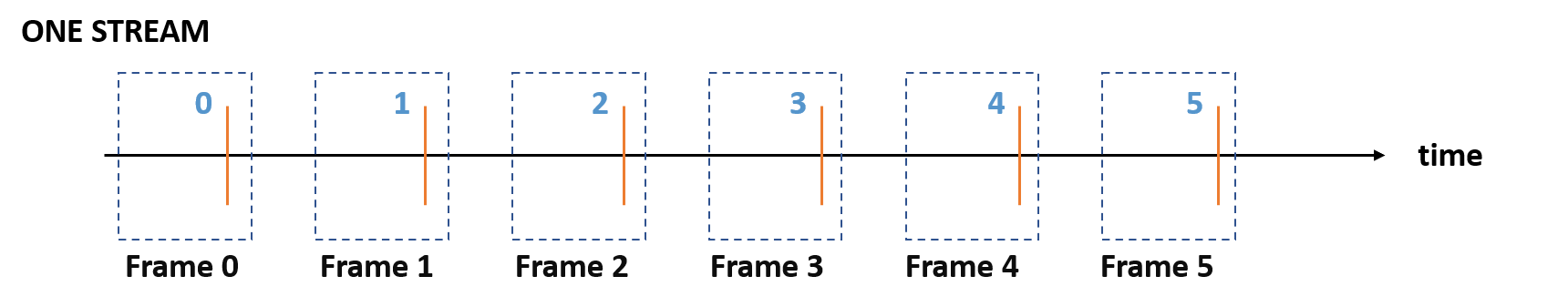1
2
3
4
5
6
7
8
{
"openlabel": {
"frames": {
"0": { ... },
"1": { ... }
}
}
}

##### Stream Frame index not coincident with Master Frame index

Though, it is possible to defined a specific frame numbering for Stream-specific frames inside the Master Frame Index (which always starts from 0). Thus, these counts are non-coincident and can reflect that the stream indexes is discontinuous or starting at a certain value.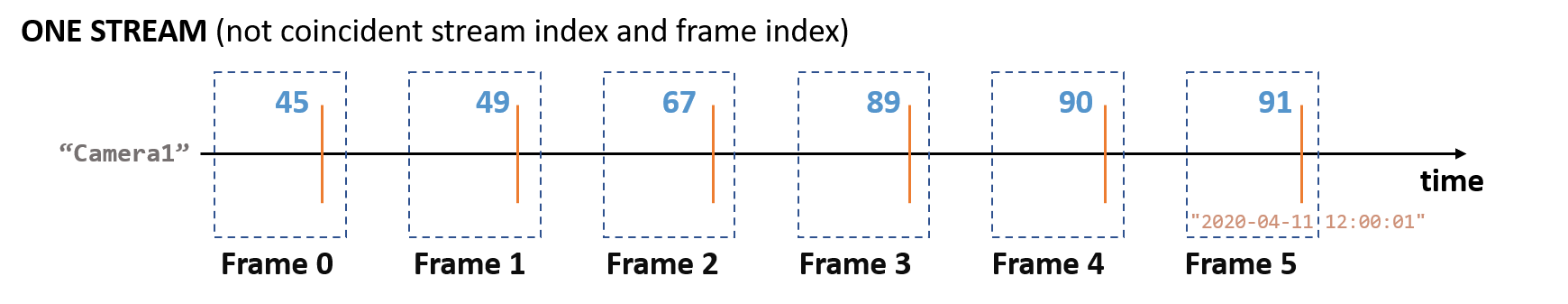1
2
3
4
5
6
7
8
9
10
11
12
13
14
15
16
17
18
{
"openlabel": {
"frames": {
"5": {
"frame_properties": {
"timestamp": "2020-04-11 12:00:01",
"streams": {
"Camera1": {
"stream_properties": {
"sync": { "frame_stream": 91}
}
}
}
}
},
}
}
}


Other properties such as timestamps can be added for detailed timing information of each stream frame.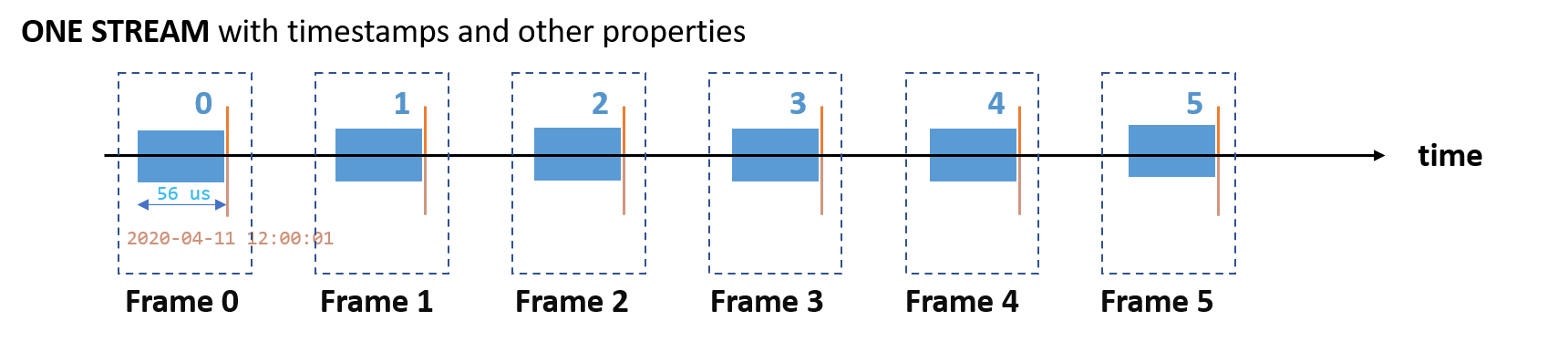1
2
3
4
5
6
7
8
9
10
11
12
{
"openlabel": {
"frames": {
"0": {
"frame_properties": {
"timestamp": "2020-04-11 12:00:01",
"aperture_time_us": "56"
}
},
}
}
}


#### 2.5.2. Multiple streams

Complex labeling set-ups include multiple streams (e.g. labels that need to be defined for different sensors).

##### Same frequency, same start and indexes

This is the fully synchronized case, where the Master Index coincides with each of the Stream indexes.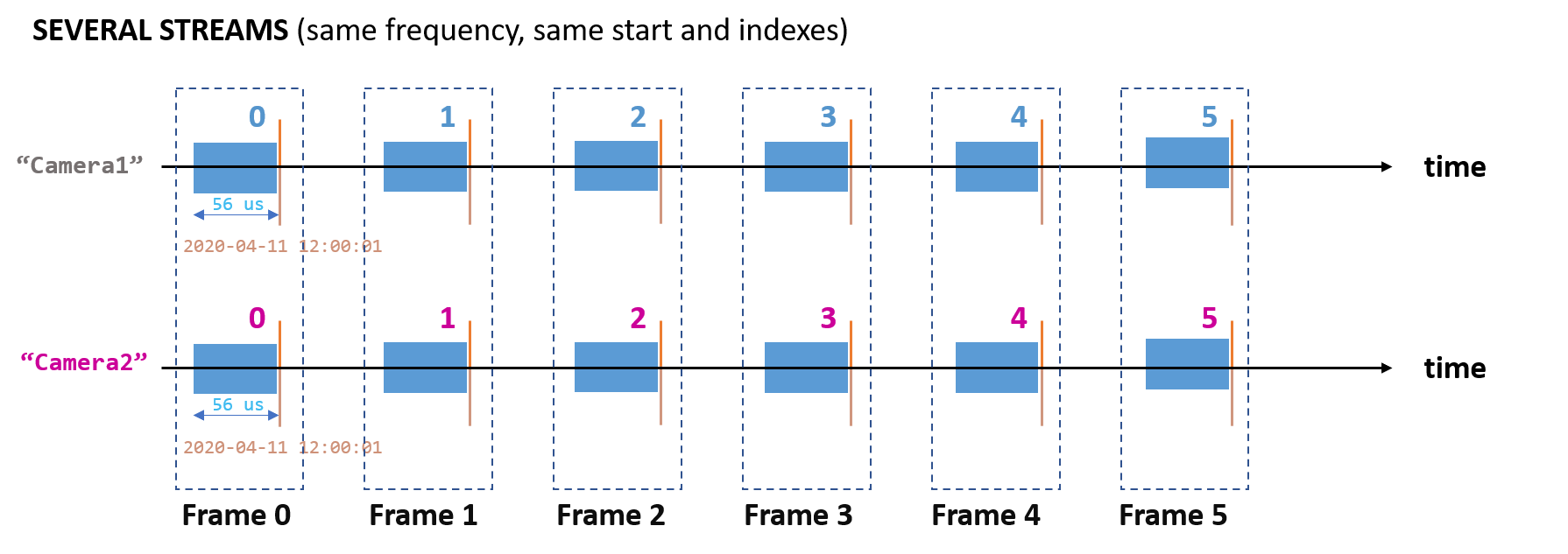##### Same frequency, different start and indexes

However, it is possible to have Stream indexes defined independently, to reflect for instance that one stream is delayed one frame (but still synced).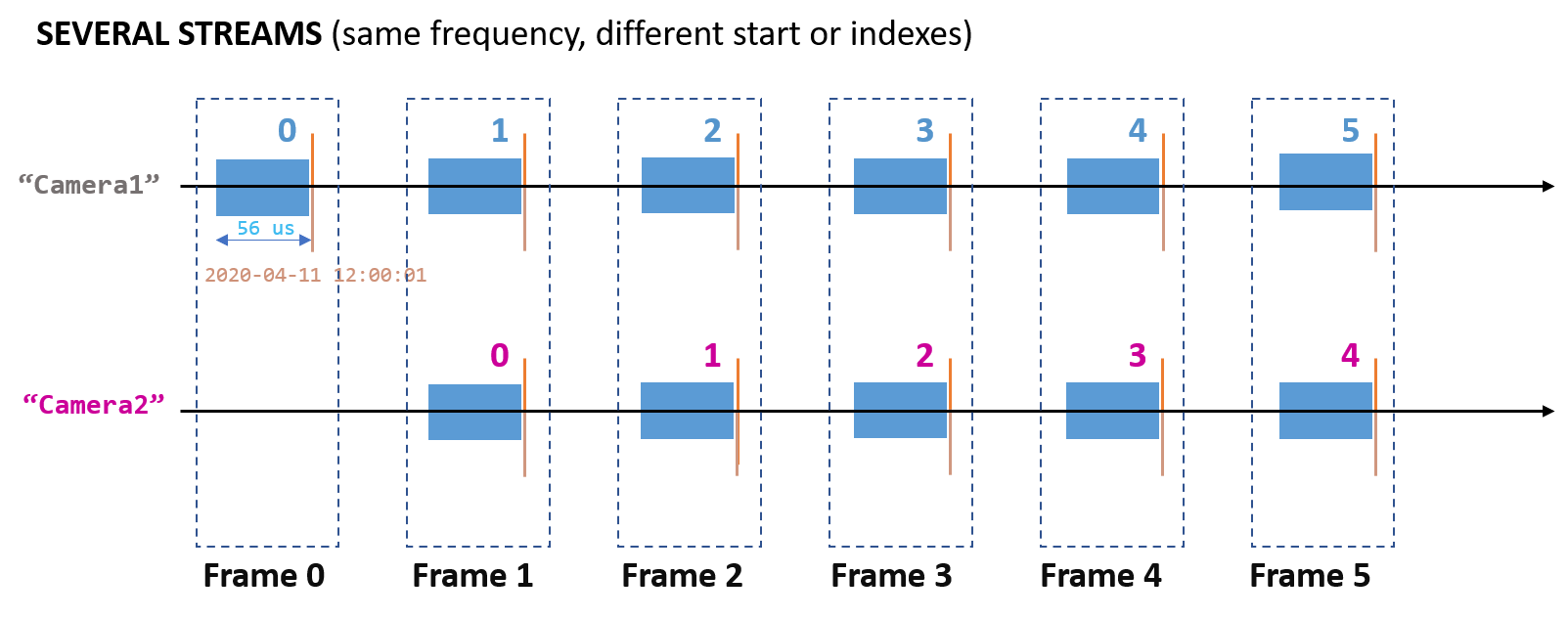1
2
3
4
5
6
7
8
9
10
11
12
13
14
15
16
17
18
19
20
21
22
23
{
"openlabel": {
"frames": {
"1": {
"frame_properties": {
"timestamp": "2020-04-11 12:00:01",
"streams": {
"Camera1": {
"stream_properties": {
"sync": { "frame_stream": 1}
}
},
"Camera2": {
"stream_properties": {
"sync": { "frame_stream": 0}
}
}
}
}
},
}
}
}


Other possible differences in syncing can be labeled, for instance jitter, by embedding timestamping information for each stream frame.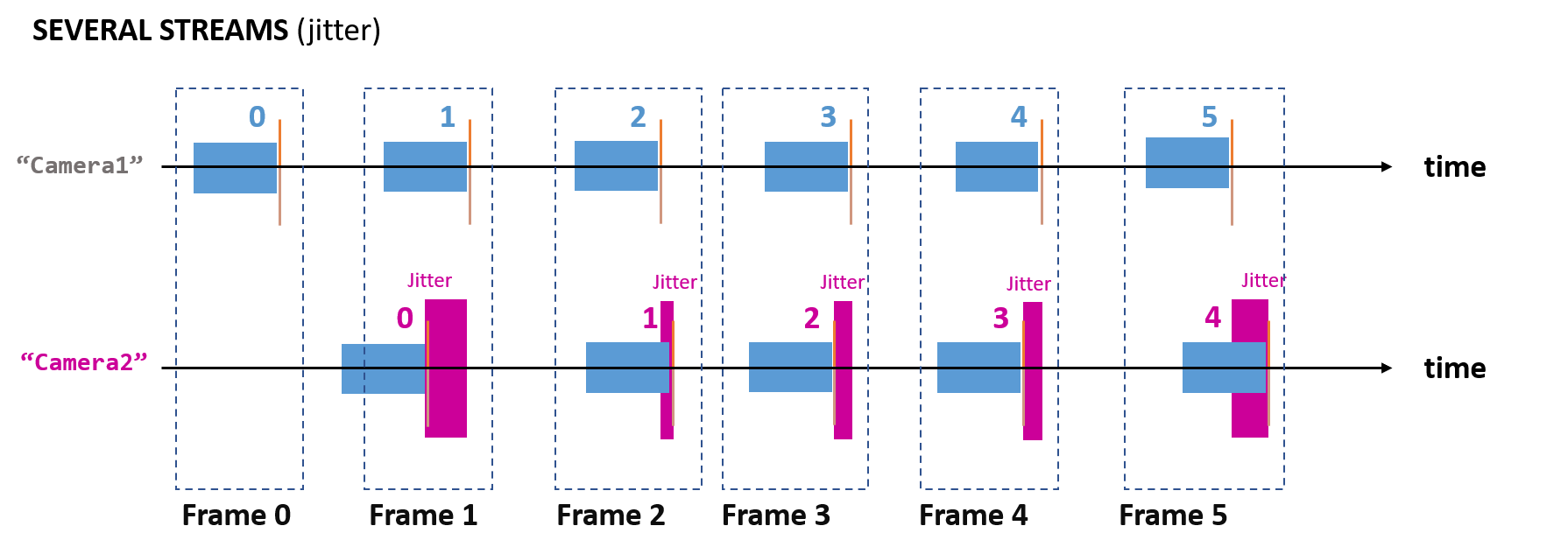##### Same frequency, constant shift

If the frame shift is known to be constant, a more compact representation is possible by specifying the shift at root stream_properties rather than on each frame (as in the previous examples):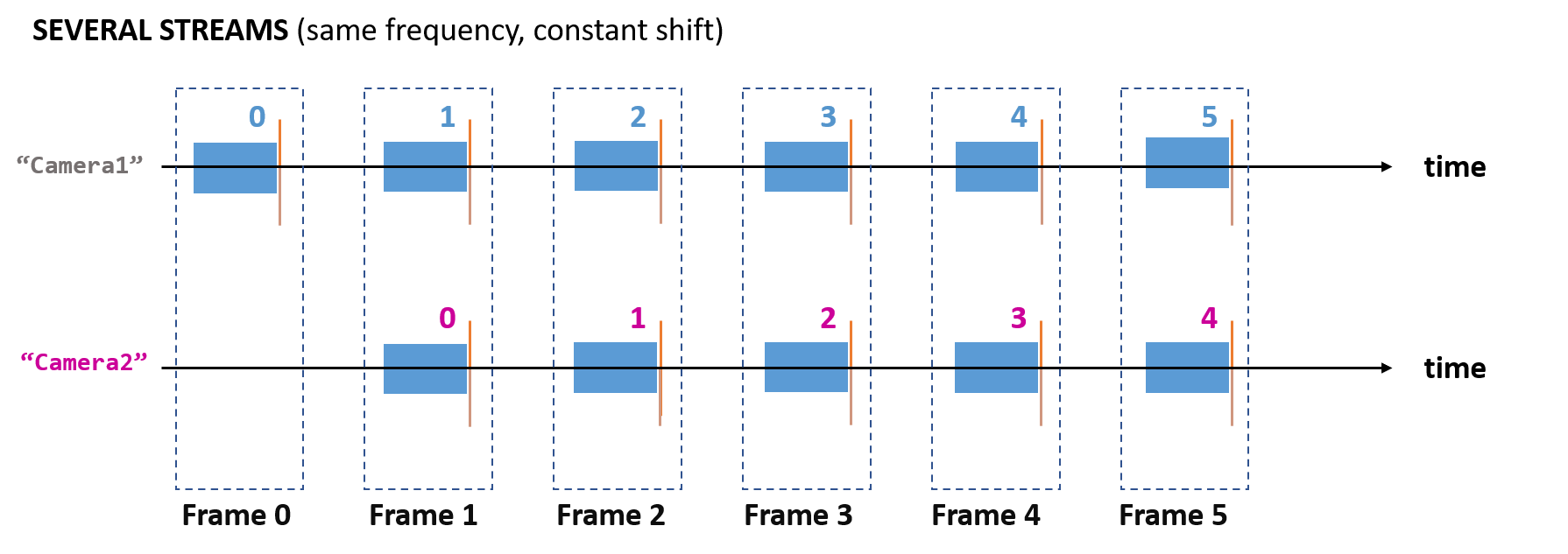1
2
3
4
5
6
7
8
9
10
11
12
13
14
15
16
{
"openlabel": {
"streams": {
"Camera1": {
"stream_properties": {
"sync": { "frame_stream": 1}
}
},
"Camera2": {
"stream_properties": {
"sync": { "frame_stream": 0}
}
}
}
}
}

##### Different frequency

Streams might represent data coming from sensors with different capturing frequency (e.g. a Camera at 30 Hz, and a LIDAR at 10 Hz). Following previous examples, it is possible to embed Stream frames inside Master frames so the frequency information is also included.

Next figures show typical configurations, where the Master Frame Index follows the "fastest" Stream (e.g. the "Camera1" Stream in the first figure), or the "slowest" (e.g. the "Lidar1" Stream in the second figure)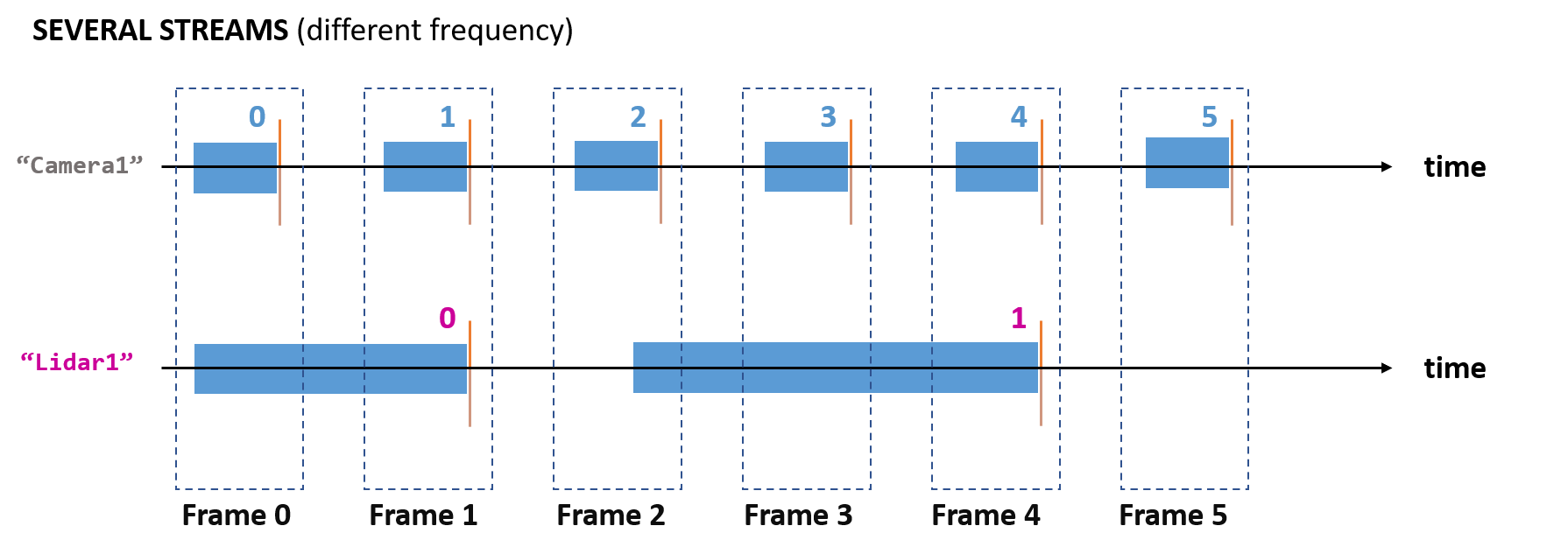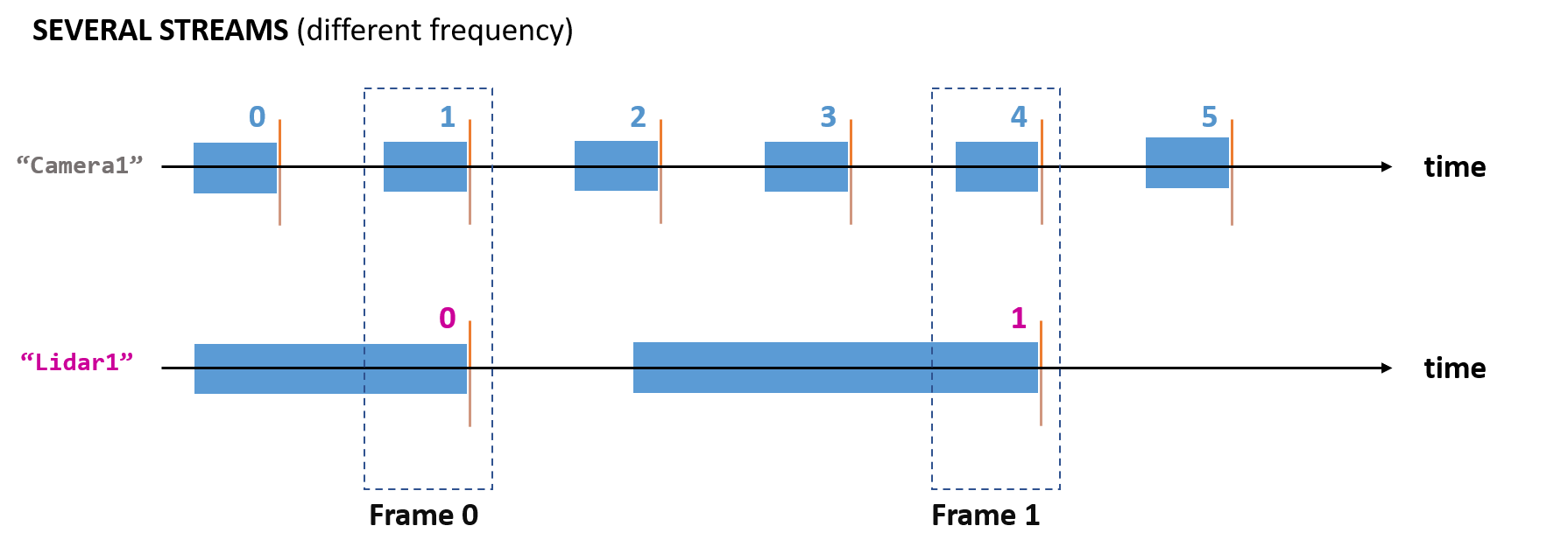#### 2.5.3. Specifying "coordinate_system" for each label

After defining the coordinate systems (see Coordinate Systems and Transforms), and the timing information as in the examples above, labels for Elements and Element data can be declared for specific coordinate systems.

Coordinate systems of specific Streams can be defined as well. This way, at each frame, the information about labels, timing, and coordinate systems is specified alltogether.

 1
2
3
4
5
6
7
8
9
10
11
12
13
14
15
16
17
18
19
20
21
22
23
24
25
26
27
28
29
30
31
32
33
34
35
36
37
38
39
40
41
42
43
44
45
46
47
48
49
{
"openlabel": {
"frames": {
"0": {
"objects": {
"0": {
"object_data": {
"bbox": [
{
"name": "shape2D",
"val": [600, 500, 100, 200],
"coordinate_system": "Camera1"
}
],
"cuboid": [
{
"name": "shape3D",
"val": [...],
"coordinate_system": "Lidar1"
}
]
}
}
},
"frame_properties": {
"streams": {
"Camera1": {
"stream_properties": {
"sync": { "frame_stream": 1, "timestamp": "2020-04-11 12:00:07"},
}
},
"Lidar1": {
"stream_properties": {
"sync": { "frame_stream": 0, "timestamp": "2020-04-11 12:00:10"}
}
}
}
}
}
},
"objects": {
"0": {
"name": "",
"type": "car",
"coordinate_system": "Camera1"
}
}
}
}


## 3. Labeling Methods

In this chapter the labeling methods for Object and scenario labeling are explaned. This chapter and all its content will be transferred to the user guide for OpenLABEL and the standrd it self.

Having a single labeling approach either for objects or scenarios is very important to asure that the datasets can be exchanged between parties, as of now, many datasets use different labeling methods and this causes the exchange or extension of datasets to be very time intensive and expensive.

### 3.1. Coordinate Systems

An OpenLABEL format data stream may contain sensor data from multiple sensors and multiple styles of label information associated with that sensor data. It needs to be clear how data from different sensors is related, how the label data relates to the sensor data and how the sensor data relates to the real world. Multiple coordinate systems with transforms between them are used to achieve this.

For example, a data stream may contain images from two forward facing cameras arranged as a stereo pair together with LIDAR return data from a single top mounted mechanically rotating LIDAR, all on a moving vehicle also containing a GNSS/INS system.

 Note that given example is more complex than an ADAS L2 applications this would be, but the given example is much simpler than a L4 AV application would.

In this example it is necessary to understand the positions and orientations of the 4 sensors (two cameras, one LIDAR, one GNSS/INS) with respect to each other and with respect to the vehicle.

The data stream may also, for example, contain label data for 2D object bounding boxes for the left camera plus 3D object bounding boxes which are a human /algorithm annotator’s best estimate of where in the world objects are based on both the stereo camera information and the LIDAR information. It is necessary to understand how the 2D and 3D labels relate to each other.

It is also necessary in many cases to understand how the sensor data and the labels relate to the real world as represented by the absolute positions and orientations the GNSS/INS system can provide, or as represented by map data. For example, it is often necessary to understand where objects are in relation to the road structure as provided by a map.

More specifically, for a camera it is desirable to understand the position of the ray of light that generated the value of each pixel in the image. This requires knowledge of the position and orientation of the camera in the world together with the positions of objects/road/etc in the world. Any distortion introduced by the camera lens also needs to be understood. For a LIDAR system, again, it is desirable to understand the point in the world which each LIDAR return is generated from. This requires knowledge of the position of the LIDAR device in the world, and the position of objects hit by the LIDAR in the world. Also since in this example the LIDAR is a relatively slow scanning mechanical device it is necessary to understand the motion of the LIDAR device through the world in order to be able to understand the positions of all the LIDAR returns relative to each other (and the world).

In general it is convenient to have a coordinate system for each sensor, together with a coordinate system for the vehicle and also a coordinate system for the world.

Sensor data is naturally stored in the coordinate system for the sensor that produced it. Labels that are specific to a sensor, e.g. 2D bounding boxes for a camera, are also naturally stored in the coordinate system of the relevant sensor. Labels that are related to world coordinates, like the fused camera and LIDAR 3D bounding box in this example are most naturally stored in the world coordinate system (that the human did the annotation in).

It may be thought that providing a fixed small number of coordinate frames, e.g. sensor(s), vehicle, world would be sufficient, however this is not the case. Different sensor set ups and different system level choices can lead to many more coordinate systems being needed with significant variation between systems.

For example, with a stereo camera system, it is usual to have a pre-processing stage that takes the ‘raw’ camera images and undistorts and rectifies them to produce a new pair of ‘rectified’ images. This removes the lens distortion and aligns the horizontal lines of both cameras (so that the rectified images fit an ideal pinhole camera model and searching for the same point in the scene in both cameras can be performed by just searching along a line). These rectified images appear to come from virtual cameras with slightly different orientations and focal lengths than the physical cameras. This difference can be represented by having an ‘image sensor’ coordinate system and a ‘(virtual) camera’ coordinate system and a transform between them. Some systems will record the ‘raw’ images and the transforms necessary to generate the rectified images (and may or may not also record the rectified images). Some systems will record only the rectified images.

For example, with a LIDAR system, it is usual to have a pre-processing stage that takes one rotation of raw LIDAR return data and converts it into a point cloud. This removes the rotating over time nature of the LIDAR scan by taking into account how the LIDAR sensor has moved (i.e. how the vehicle has moved) over the time taken for the LIDAR to make one rotation. This ‘untwisting’ process generates a point cloud in a coordinate system that is fixed in the world. In some systems the point cloud is in a coordinate system that is locked to where the LIDAR was at the start (or end) of the scan; in other systems it is in a coordinate system that is fixed in the world but can drift over time relative to a map (an ‘odom’ type coordinate system); and in some systems it is in a coordinate system that is absolutely fixed in the world (a GNSS or map type coordinate system).

Another reason different systems may use different coordinate systems is that the maps they use may be different. For example, some systems may use GNSS type coordinates (an elliptical coordinate system); some systems may use UTM coordinates (rectangular coordinates that are appropriate for local areas of the earth); and some systems may use country specific mapping systems (such as the UK Ordinance Survey map coordinates). Other systems may use proprietary maps such as those created by dense LIDAR surveys of an area together with a LIDAR localisation method.

Yet another reason that different systems may use different coordinate systems is that they may have made different system level choices for how to represent motion of the vehicle through the world. For example, many systems use the model that the ROS (Robot Operating System) middleware uses where the chain of transforms includes the ‘odom’ coordinate system which is a coordinate system that is approximately fixed in the world but can drift over time. In this model the transform sequence is typically: sensor → vehicle → odom → map → earth. In this case the system generates its own idea of motion (odometry) through the world using local sensor data like camera or inertial sensors, then this ‘odom’ coordinate system is localised by placing it on a map using some global sensor data like GNSS. The benefit of this system is that it can gracefully handle situations where there is a loss of global sensor data such as when a vehicle enters a tunnel and loses GNSS signal. In this case the vehicle will be able to detect its movement through the tunnel, but only approximately and over time the vehicle’s idea of its position on a map will drift from its actual position on a map, then when the vehicle emerges from the tunnel it will regain GNSS signal and be able to correct its idea of position on the map. This is represented by the vehicle motion being smooth in the odom coordinate system, but there being discontinuous changes of the transformation between the odom and map coordinate systems.

Yet another system level choice for different systems using different coordinate systems might be choices like whether to compensate for tilt of the road. Some systems may have an additional coordinate system (at some point in the transform sequence) that is referenced to ‘down’ as detected by using accelerometers (with compensation for acceleration due to motion).

For all these reasons the OpenLABEL standard provides a method to describe an arbitrary number of coordinate systems and a method to describe the transforms between those coordinate systems.

However, despite the ability to describe an arbitrary set of coordinate systems, there are some coordinate systems that are commonly used in many systems and so are defined by the standard. The coordinate systems with fixed definitions include: “vehicle-iso8855”, “odom”, “map-UTM”, “geographic-wgs84”. Whenever these names are used for a coordinate system, they shall have the meaning defined in the standard.

It is also important to note that the transformations between coordinate systems can vary over time. In the example above the odom to map transform varies as the vehicle emerges from the tunnel. Even transforms that might appear fixed, because they are rigidly connected like the transformation from camera to vehicle, can in fact vary over time. For example, a camera system may have a dynamic re-calibration system which may change the transformation between the camera sensor coordinate system and the (virtual) camera coordinate system, thus changing the transform between the camera and the vehicle.

The OpenLABEL standard therefore provides a method to describe transforms which are fixed for all time, which vary occasionally (at a specific frame), and which vary continuously (every frame).

Concepts

The key concepts are:

• Coordinate-system – a way of using one or more numbers, ‘coordinates’, to specify the location of points in some space. E.g. the 2D position of a pixel within an image or the 3D position of a LIDAR return point in the world relative to the vehicle’s rear axle. Coordinate systems are often, but by no means always, 3D right-handed cartesian systems.

• Transform – a transformation allowing the coordinates one coordinate-system to be converted into coordinates in another coordinate-system such that they represent the same point in space. A transform always has two coordinate-systems associated with it, a source and a target.

Each coordinate-system has a textual name and a uid that is used to reference it within the OpenLABEL JSON data stream. A small number of names are reserved and refer to pre-defined coordinate systems specified in the standard. All other names are user defined. Each coordinate-system is defined by either being associated with a sensor, or by being the source or target of a transform, where there is a sequence of such transforms that ends in either a sensor or a pre-defined coordinate system.

Each transform is one of a fixed number of types. The types supported are:

• camera-transform – a projective transform describing how a point in the real world is translated into a pixel on the camera sensor. Usually split into several components, intrinsics, distortion coeficients and extrinsics. [TODO, should we support multiple types of camera-transform with different complexities of distortion model?]

• cartesian-transform – a 3D to 3D transform offering a change of origin, scale and rotation. Represented as a matrix and a quaternion.

• geospatial-transform – a transform from a 3D Cartesian coordinate system into an ellipsoidal GNSS style coordinate system. E.g. from map-UTM to WGS84 latitude, longitude, altitude.

 [ Note that the current JSON schema does not seem to allow for arbitrary numbers of coordinate systems each having their own name. It seems to assume the existence of just vehicle and world coordinate systems and then describes transforms from sensors to these, and a transform between vehicle and world coordinates. I believe we should change this to allow an arbitrary number of coordinate systems with transforms between them. I suggest a coordinate-system in the JSON should be a string and there should be a way to associate a coordinate system with a sensor. I suggest a transform in the JSON stream should include: source-coordinate-system, target-coordinate-system, transform-type, transform parameters (as arrays). ]

Pre-defined coordinate systems

The following coordinate system names are predefined, and wherever used have the following meaning: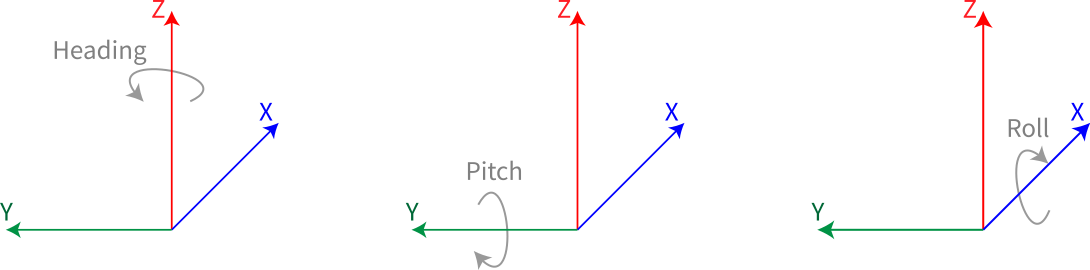Figure 1. coordinate systems with heading, pitch and roll
• "vehicle-iso8855” – a right-handed coordinate system with origin at the centre of the rear axle projected down to ground level. Note the origin is attached to the rigid body of the vehicle (not actually an axle that has suspension components between it and the vehicle body). It is at ground level with the vehicle nominally loaded, depending on the actual loading it may in fact be above or below ground level. Similarly, the axis pointing forward may actually point slightly upwards or downwards relative to ground level depending on the front to back loading of the vehicle. The x axis is forward, the y axis to the left and the z axis upwards. See the ISO 8855 specification.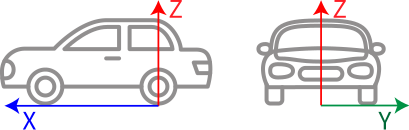Figure 2. Vehicle coordinate system, ISO 8855
• “odom” – a 3D cartesian coordinate system that is approximately fixed in the world. The transform between the vehicle-iso8855 coordinate system and this one is guaranteed to be continuous (i.e. will vary smoothly over time). Note that the transform between odom and map-UTM is may be discontinuous (i.e. there may be sudden jumps in the value of the transform). The odom origin is often the starting point of the vehicle at the time the system is switched on. See the ROS documentation.

• “map-UTM” – a 3D cartesian coordinate system useful for mapping moderately sized regions of the earth. It is locked to the earth and is a set of slices of flat coordinates that cover the earth. See the UTM specification. ]

• geospatial-wgs84” – 3D ellipsoidal coordinate system used for GNSS systems. I.e. latitude, longitude, altitude. It is fixed to the earth (ignoring continental drift etc ) and covers the entire earth. See the various GPS specifications.

Typical transform trees

There are several sets of coordinate systems (blue boxes) and transforms (blue lines) between them that are commonly used.

For example, a ROS based system with the sensors described in the example system in the introduction might have the following transform tree: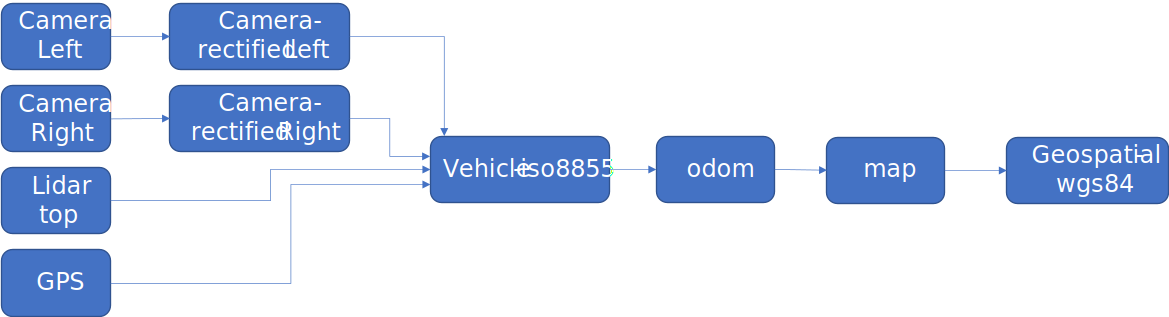A set of data captured from a dash-cam (single camera plus GPS) might look like: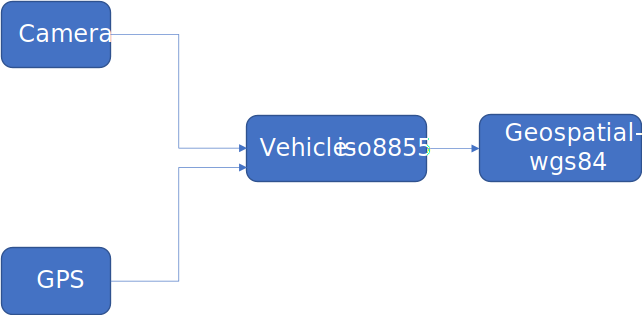A single camera with no other data, with with the movement of the camera deduced by structure from motion, might look like: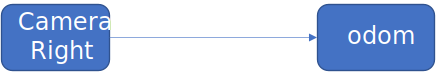### 3.2. Geometries for labeling

When labelling objects with in data streams different geometries are necessary. depending on the typ of sensor stream either 2D or 3D geometries are needed. Therefore the OpenLABEL Standard will provide a set of primitives that can used to label objects and areas in the sensor streams.

The format described in chapter 1 supports the following geometry types :

• bbox: a 2D bounding box

• rbbox: a 2D rotated bounding box

• cuboid: a 3D cuboid

• point2d: a point in 2D space

• point3d: a point in 3D space

• poly2d: a 2D polygon defined by a sequence of 2D points

• poly3d: a 3D polygon defined by a sequence of 3D points

• area_reference: a reference to an area

• line_reference: a reference to a line

• mesh: a 3D mesh of points, vertex and areas

#### 3.2.1. Point

A point in the two dimensional space has two coordinates: x and y in the three dimensional space a point is defined by three coordinates x, y and z.

In the below example a 2D point is defined at the coordinates x=100 and y=100.

"point2d": {
"name": "2D_point",
"val": [100,100]
}

In the below example a 3D point is defined at the coordinates x=100 and y=100 and z=50.

"point3d": {
"name": "3D_point",
"val": [100,100,50]
}

#### 3.2.2. Line

A Line is a basic element which is defined by two points. Defining a line by using two points will make reduce differences when computing the lines on different systems with different implementations. When defining a line with a starting point and a length the endpoint of the line has to be calculated. Depending on the system the results can differ slightly.

"line_reference": {
"name": "line",
"val": ["TODO: definition of the line"]
}

#### 3.2.3. Boxes

Boxes are used as a very basic tool to label objects. usually a box is placed around an object and enclosed this completely. For many use cases the exact outline of an object is not necessary and therefor would only cost computational power. to avoid this boxes can be used. For example when labeling a parking car, the exact outline of the car is not needed as the car would not pass so close to the parking car as that the exact outline would be beneficial for the path calculation. In OpenLABEL there are two kinds of boxes:

• 2D Boxes

• 3D Boxes also called cuboids

Example for and 2D bounding box:

"bbox" : [{
"name" : "",
"stream" : "CAM_LEFT",
"val" : [296.74, 161.75, 158.48, 130.62]
}
],

Example for and 3D bounding box or cuboid:

"cuboid" : [{
"name" : "",
"val" : [14.44, 4.55, -0.2, 0, 0, -2.11, 1.82, 4.43, 2.0]
}
]

ASAM OSI definition for a 2d or 3d box: Allowed number of referenced points: 2 or 3

Allowed number of referenced points = 2: first and third corner of the box. Box is aligned horizontal resp. vertical.

Allowed number of referenced points = 3: first, second and third corner of the box. fourth corner is calculated by first+third-second corner.

#### 3.2.4. Polygons

a more complex primitive is a polygon, in OpenLABEL there are two types of polygons. Polygons can be very useful to label more complex outlines when necessary.

• 2D Polygons

• 3D Polygons

ASAM OSI definition for a polygon

Allowed number of referenced points: 3 .. n

Polygon is defined by the first, second, third and so on points. The polygon shape is closed (last and first point are different).

"poly2d" :[{
"name" :"2d-polygon"
"val" : ["a","b","c","d"]
}]

it is also possible to create 3d Polygons for 3 dimensional data e.g. Lidar point clouds

"poly3d" :[{
"name" :"3d-polygon"
"val" : ["..."]]
}]

### 3.3. Spatial Rotation

There are several ways to describe spatial rotations, each of which has its own advantages and disadvantages. This chapter discusses these methods as well as their differences.

#### 3.3.1. Rotation matrices

A rotation matrix is a 3x3 matrix which consists of orthogonal unit vectors, i. e. it is an orthonormalized matrix.

The multiplication of rotation matrices equals the concatenation of rotations and thus yields rotations matrices. However, because of floating point errors, the resulting matrix needs to be orthonormalized. Therefore, the Gram-Schmidt process can be applied.

#### 3.3.2. Euler Angles

A rotation can be described using Euler Angles (roll, pitch, yaw).

#### 3.3.3. Quaternions

To understand quaternions, it might help to remember complex numbers, their properties and the reason why they describe rotations in two dimensional space.

##### Complex Nnumbers

Complex numbers, introduce the imaginary value $$i$$ with the property $$i^2=-1$$. A complex number $$a+bi$$ can be represented as a point in a 2d-coordinate system.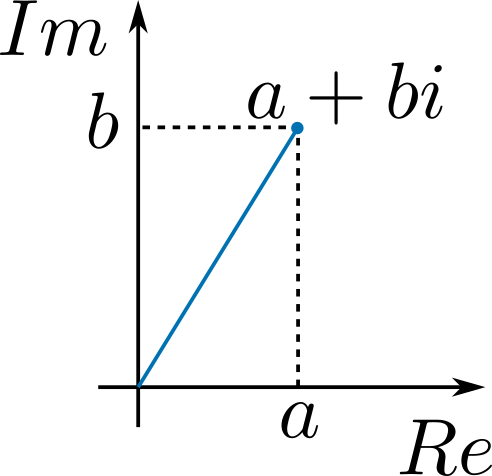With $$i^2=-1$$, the multiplication of two complex numbers yields a rotation around the origin (and a multiplication of their radii).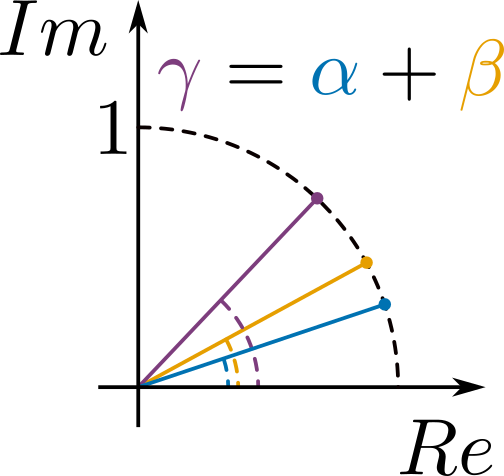##### Quaternions

Quaternions use a similar mathematical concept as complex numbers. Therefore, we introduce three imaginary values $$i, j, k$$ and the following axioms in form of a multiplication table:

 * i j k i -1 k -j j -k -1 i k j -i -1
• $$i^2=j^2=k^2=-1$$

• $$i*j=k, \quad\quad j*k=i, \quad\quad k*i=j$$

Considering a 3d-coordinate system where each imaginary value is associated a unique dimension, these axioms already yield the rotation around certain axis. The following picture shows a primitive example. To rotate vectors by a quaternion, vectors are represented as quaternions by linear combination of the i, j, and k with the same coefficients as the corresponding unit vectors. To rotate the $$i$$-vector around the latexmk:[j]-vector, simply multiply it with j. The result is the latexmk:[k]-vector. Hence, $$i$$ was rotated around $$j$$ by 90 degrees.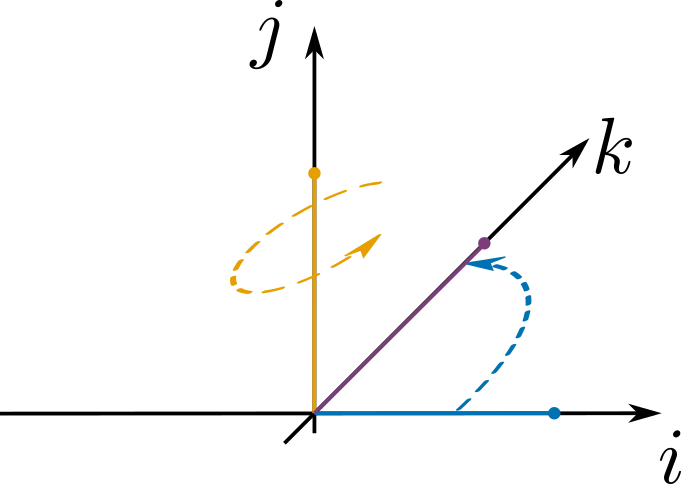In general, quaternions can be thought of as rotations around a certain axis with a certain angle.

##### The math behind quaternions
• A quaternion $$q=w+xi+yj+zk$$ is normalized if its norm $$n(q)$$ equals $$1$$, where

$n(q) = \sqrt{w^2 + x^2 + y^2 + z^2}.$
• The conjugate of $$q$$ lets the "rotation axis" point into the other direction.

$\bar q = w-xi-yj-zk$
• Quaternions form an algebraic field, thus addition, subtraction, multiplication, and division are defined.

• The neutral element regarding multiplication is $$\quad 1 = 1 + 0i + 0j + 0k.$$

• The inverse regarding multiplication is

$q^{-1} = \frac{\bar q}{n(q)^2}.$
• A vector $$p=(p_1, p_2, p_3)$$ is rotated by q via $$q' = q*(0 + p_1i + p_2j + p_3k)*\bar q$$.

• The unit quaternion which does the rotation around a unit vector considered as axis $$u=(u_1, u_2, u_3)$$ with an angle $$a$$ is constructed via

$q = cos\left(\frac w 2\right) + sin\left(\frac x 2\right) u_1i + sin\left(\frac y 2\right) u_2j + sin\left(\frac z 2\right) u_3k$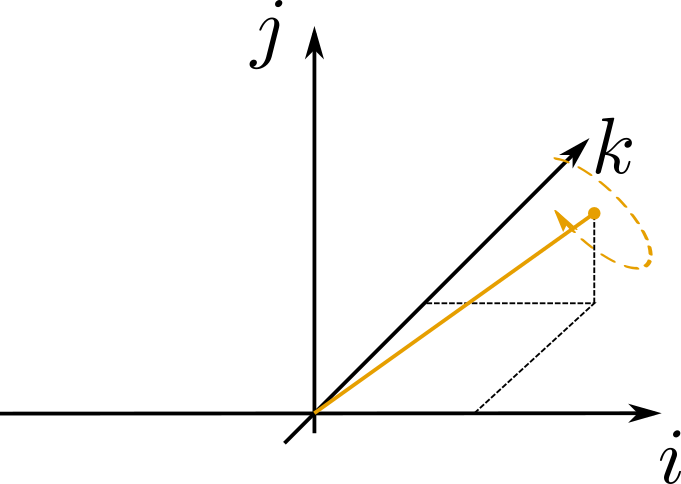##### Comparison between rotation representations

Euler angles have the advantage of being easy to understand and explain. However, they are the most difficult representation for computers since they first have to be transformed to rotation matrices or quaternions before being applied. Euler angles also suffer from the gimbal lock, which is a state of rotation where all further rotation degenerates into having only two degrees of freedom instead of three. However, Euler angles don’t need to be normalized as do quaternions and rotation matrices.

For these reasons, the following table only compares the computation time and storage requirements of quaternions and rotation matrices.

Quaternion Rotation Matrix

Storage

4(3)

9

Operations for chaining rotation

24

45

Operations for vector rotation

30

15

Normalization

cheap (normalize)

expensive (Gram Schmidt process)

The fourth component of a unit quaternion can always be derived from the other three, i.e. quaternions need as few storage as Euler angles. However, this would always need an extra computation step.

The normalization of a quaternion is quite simple since it just needs to be divided by its norm. On the other hand, the normalization of rotation matrices is quite complex, since it is not just the length of all column vectors which need to be normalized, but also the right angle between all column vectors. This can be achieved with the Gram Schmidth process which requires much more computation steps than just one normalization.

For the reasons described above, we consider unit quaternions stored with four floating point values to describe rotations of objects such as bounding boxes or frames of coordinate systems.

##### Helper functions for quaternions

The following function returns the angle, a quaternion would rotate a vector around its rotation axis.

function angle( Quaternion q )
q = n( q )
if( q.z >= 0 )
return 2*acos( q.w )
else
return -2*acos( q.w )

The axis of a quaternion can be retrieved as well. If the rotation angle is (very close to) zero, the rotation axis is ambiguous since it could be any rotation axis. In this case the function returns a default axis (e.g. the last unit vector).

function axis( Quaternion q )
if( n(q) < epsilon )
return ( 0, 0, 1 )
else if( q.z >= 0 )
return 1/n(q) * ( q.x, q.y, q.z )
else
return -1/n(q) * ( q.x, q.y, q.z )

Please note, that the quaternions $$q$$ and $$-q$$ represent the same rotation. This is easy to see when imagining the quaternion as a rotation around an axis: Negating the axis simply switches the rotation direction. However, negating the rotating angle too cancels out the change of rotation direction.

Also, we normalized the rotation axis in such a way that it always points up (i. e. in the positive $$z$$-axis).

##### Transformation between rotation representations

Quaternion to rotation matrix:

Let $$q=w+xi+yj+zk$$ be a normalized quaternion. Then, the corresponding rotation matrix is

$M(q) = \begin{pmatrix} x^2-y^2-z^2 + w^2 & 2*(x*y - z*w) & 2*(x*z + y*w) \\ 2*(x*y + z*w) & -x^2 + y^2 - z^2 + w^2 & 2*(y*z-x*w) \\ 2*(x*z - y*w) & 2*(y*z + x*w) & -x^2 -y^2 + z^2 + w^2 \end{pmatrix}$

Rotation matrix to quaternion:

On the other hand, a rotation matrix $$m$$ can be transformed into a quaternion:

$Q(m) = \frac 1 2 \sqrt{1 + trace(m)} + \frac{(m_{2, 1} - m_{1, 2})}{ 2 \sqrt{1 + trace(m)} }i + \frac{(m_{0, 2} - m_{2, 0})}{ 2 \sqrt{1 + trace(m)} }j + \frac{(m_{1, 0} - m_{0, 1})}{ 2 \sqrt{1 + trace(m)} }k$

with $$trace(m) = m_{0,0} + m_{1,1} + m_{2,2}.$$

Quaternion to Euler angles:

The Euler angles roll ($$\gamma$$), pitch ($$\beta$$), and yaw ($$\alpha$$) can be retrieved from a quaternio $$q$$ as follows:

$\alpha(q) = \text{atan2}\left( 2*q.y*q.w - 2*q.x*q.z, 1-2*q.y^2-2*q.z^2 \right) \\ \beta(q) = \text{asin}\left( 2*q.x*q.y + 2*q.z*q.w \right)\\ \gamma(q) = \text{atan2}\left( 2*q.x*q.w - 2*q.y*q.z, 1-2*q.x^2-2*q.z^2 \right)$

However, there are two exceptions at the poles: If $$q.x*q.y+q.z*q.w = 0.5$$, then

$\alpha(q) = 2*\text{atan2}( q.x, q.w ) \\ \beta(q) = \frac\pi 2 \\ \gamma(q) = 0$

and if $$q.x*q.y+q.z*q.w = -0.5$$, then

$\alpha(q) = -2*\text{atan2}( q.x, q.w ) \\ \beta(q) = -\frac\pi 2 \\ \gamma(q) = 0$

Euler angle to quaternion:

A different order of application of roll, pitch, and yaw yields different overall rotations. We suppose that roll ($$\alpha$$), pitch ($$\beta$$), and yaw ($$\gamma$$) are applied in the order $$\gamma, \beta, \alpha$$. Then, the overall rotation is represented by the following quaternion:

$Q(\alpha, \beta, \gamma) = \cos\frac\gamma 2 \cos\frac\beta 2 \cos\frac\alpha 2 - \sin\frac\gamma 2 \sin\frac\beta 2 \sin\frac\alpha 2 \\ +\left(\sin\frac\gamma 2 \sin\frac\beta 2 \cos\frac\alpha 2 + \cos\frac\gamma 2 \cos\frac\beta 2 \sin\frac\gamma 2 \right)i \\ +\left(\sin\frac\gamma 2 \cos\frac\beta 2 \cos\frac\alpha 2 + \cos\frac\gamma 2 \sin\frac\beta 2 \sin\frac\gamma 2 \right)j \\ +\left(\cos\frac\gamma 2 \sin\frac\beta 2 \cos\frac\alpha 2 + \sin\frac\gamma 2 \cos\frac\beta 2 \sin\frac\gamma 2 \right)k$
##### Standards

Rotations of bounding boxes, point clouds, or other three-dimensional objects shall be represented using quaternions. A quaternion shall be notated using four floating point numbers $$q=(w,x,y,z)$$ of double precision where the first component shall represent the real part of the quaternion (TODO: reference to the user guide). Although rotations are represented by normalized quaternions which only have three degrees of freedom, the quaternion shall be written using four numbers such that the norm acts as a checksum.

Whenever the $$z$$-component of a quaternion is negative, the quaternion should be negated (i. e. consider $$-q$$) in order to avoid ambiguities. The angle of rotation should always be considered in the interval $$[-\pi, \pi$$].

The rotation axis of a quaternion $$q$$ representing a rotation with no angle (i. e. it corresponds to the identity function) should be the $$z$$-axis, i. e. $$axis(q)=(0, 0, 1)$$.

### 3.4. Bounding Boxes

Description:

Bounding Boxes are used to label objects and entities detected by sensors mounted e.g on a vehicle. There are different types of bounding boxes 2D/3D.
Ususally the primary sensor for 2D bounding boxes is the camera.
Ususally the primary sensor for 3D bounding boxes is the lidar or radar.

Using bounding boxes is a cost and time efficient way to label data. It is easiery to draw boxes over detailed "painting" of areas in the data. Data sets that are labeled with bounding boxes are also cheaper to process in terms of processing power and use less space in storage.

Depending on the target Machine Learning Network datasets labeled with bounding boxes are mandatory.

#### 3.4.1. 3D Bounding Boxes / Cuboids

Description: A 3D bounding box provides a rough size estimation of an object in height, width and length, along with its position and rotation in 3D space. A 3D bounding is defined in as rectangular cuboid (from now on, cuboid), having 9 degrees of freedom:

• 3 for position (x,y,z)

• 3 for rotation (rx, ry, rz) = (roll, pitch, yaw)

• 3 for size (sx, sy, sz) = (length, width, height)

To have an unambiguous representation of the cuboid in 3D space, it is necessary to declare the convention that provides meaning to these rotation and size magnitudes. Therefore, a cuboid is defined as a 9-dimensions vector:

$$c=(x, y, z, r_x, r_y, r_z, s_x, s_y, s_z)$$

• $$(x, y, z)$$ is the position of the center point of the cuboid, in meters;

• $$(r_x, r_y, r_z)$$ are the (improper) Euler angles associated to the x, y and z-axes, in radians. These angles shall be expressed as intrinsic, active (alibi) rotations so that a transformation built with these angles and position can be used to change the cuboid as a rigid body with respect to a certain coordinate system. The convention is that $$r_x$$ =roll, $$r_y$$ = pitch, and $$r_z$$ = yaw, to follow usual industrial standards. Rotations shall be applied Z→Y'→X''.

• $$(s_x, s_y, s_z)$$ are the dimensions of the cuboid, in meters. Note $$s_x$$ expresses "length", $$s_y$$ "width", and $$s_z$$ "height", although these terms are only meaningful depending on the observer coordinate system and conventions. In this document, the ISO 8855 is taken as example, where x-axis is the longitudinal axis (thus x="length"), y-axis is the transversal axis (y="width"), and the z-axis is the vertical axis (z="height"), so a ground plane in reality will coincide with z=0.

 The order of rotations shall be Z→Y'→X'', and must be followed, otherwise, the cuboid rotation will be different, as there are multiple Euler angles to express the same rotation, and consequently, different order execution of Euler angles produce different rotations.
 The rotations on axes Z, Y, and X must be intrinsic. That means that after each rotation, the next rotation is applied on the new axes of the object, which have been rotated after each step. This is commonly notated as Z→Y'→X'' contrary to Z→Y→X which assumes that all rotations are expressed with respect to the first/original axes.

Examples: Simple examples of the described concepts can be seen in the following images.